## ARIMA and SARIMA in Rstudio

There are several packages available for estimating the ARIMA and SARIMA in Rstudio. Autoregressive Integrated Moving Average (ARIMA) and Seasonal Autoregressive Integrated Moving Average (SARIMA) models are often used for forecasting purposes. These time series models often provide good forecasting performance.

To understand the estimation procedure of these models, you can check out the posts on ARIMA Estimation and Model Selection, and Seasonality and Seasonal-ARIMA models.

## Application of ARIMA and SARIMA in Rstudio

knitr::opts_chunk$set(echo = TRUE) library(tidyverse) library(magrittr) library(stargazer) library(haven) library(skedastic) library(car) library(datasets) library(tseries) library(forecast) tsdata <- datasets::AirPassengers view(tsdata) Decompose The “decompose” function can be useful in extracting information about the time series. This function attempts to decompose the series into its components: trend, seasonality and the random component. Additionally, we can also choose the seasonality to be additive or multiplicative. This decomposition can provide valuable insights when choosing the order of ARIMA or SARIMA models. plot(tsdata)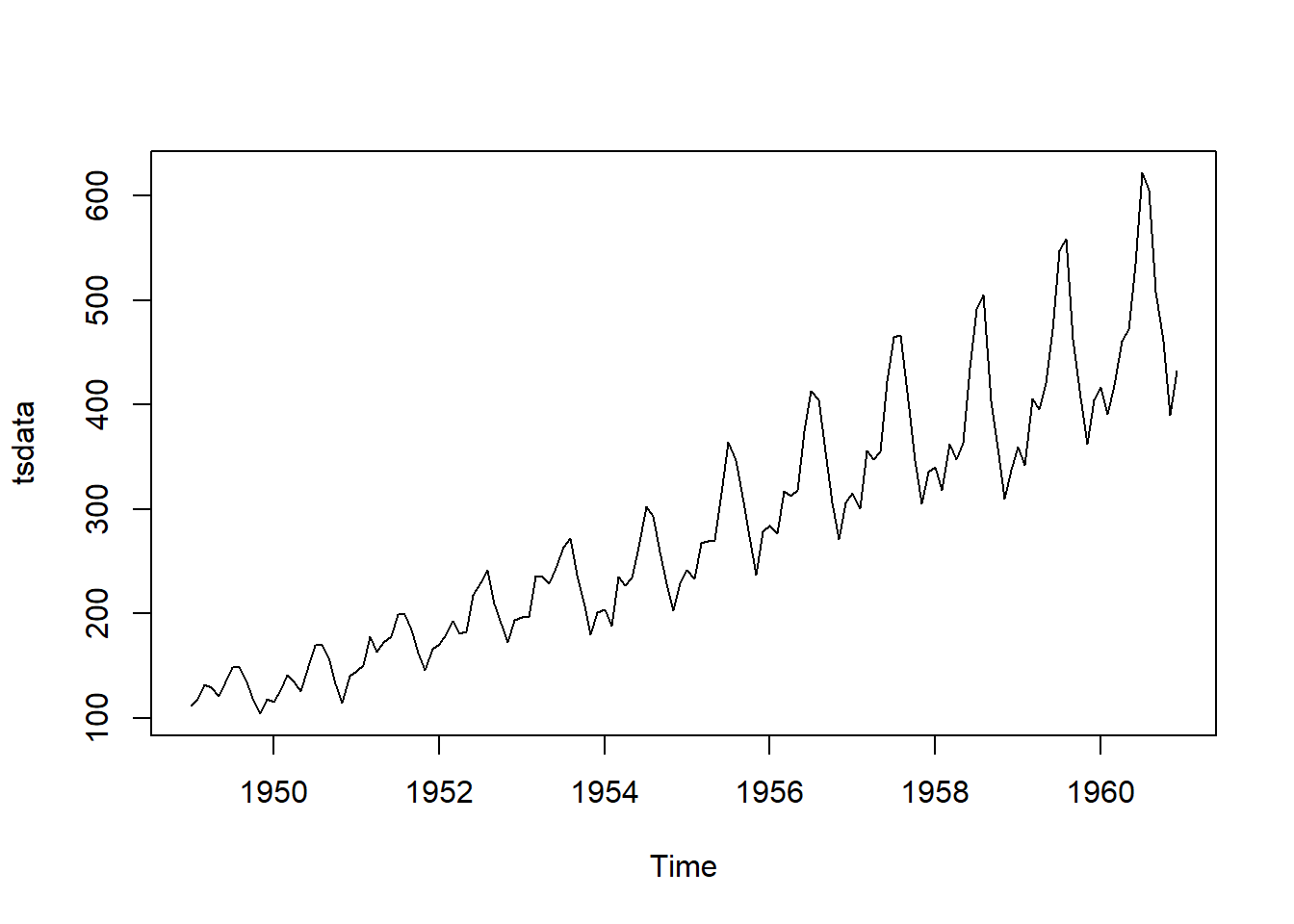dec_add <- decompose(tsdata, type = "additive") dec_mul <- decompose(tsdata, type = "multiplicative") plot(dec_add)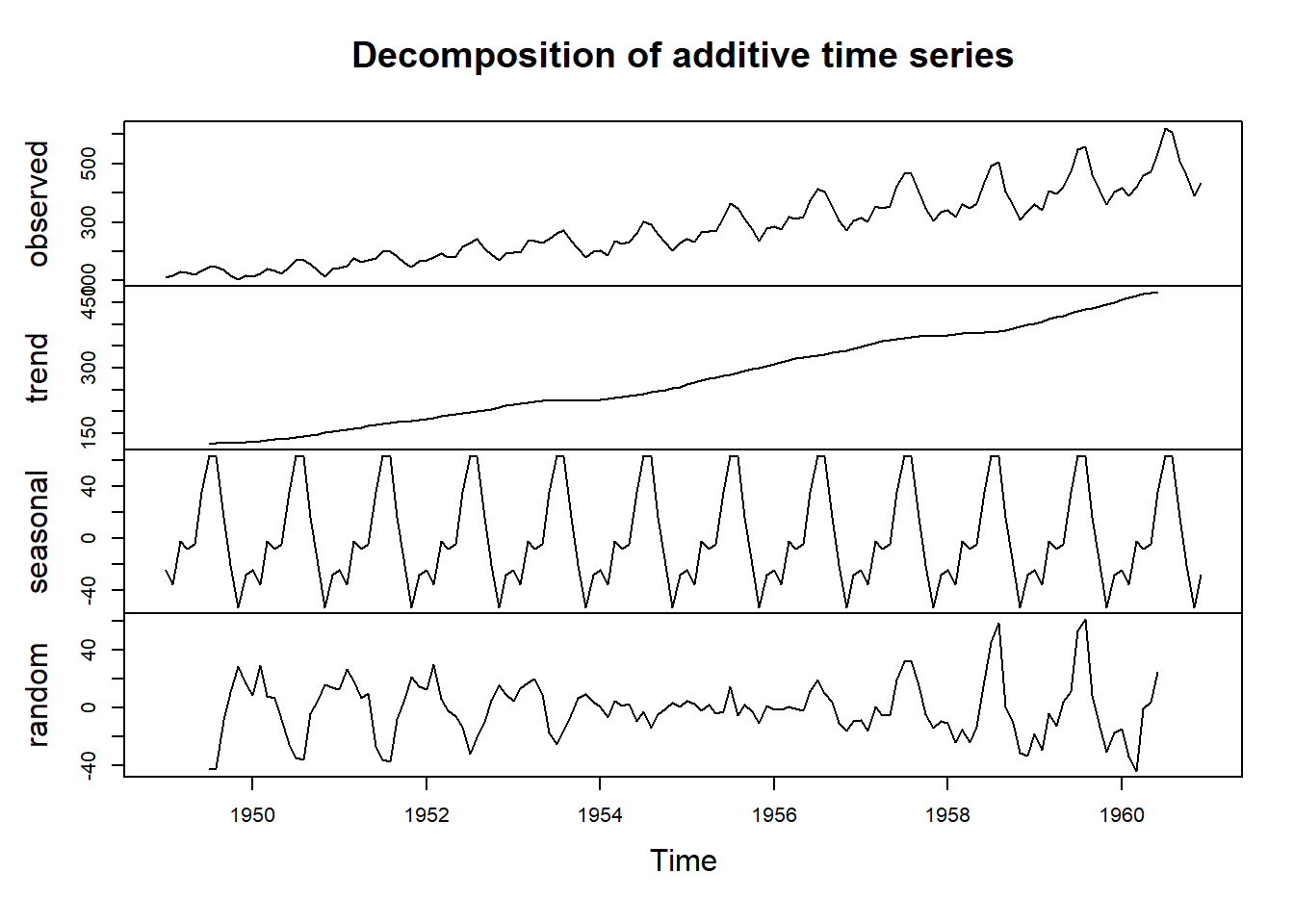plot(dec_mul)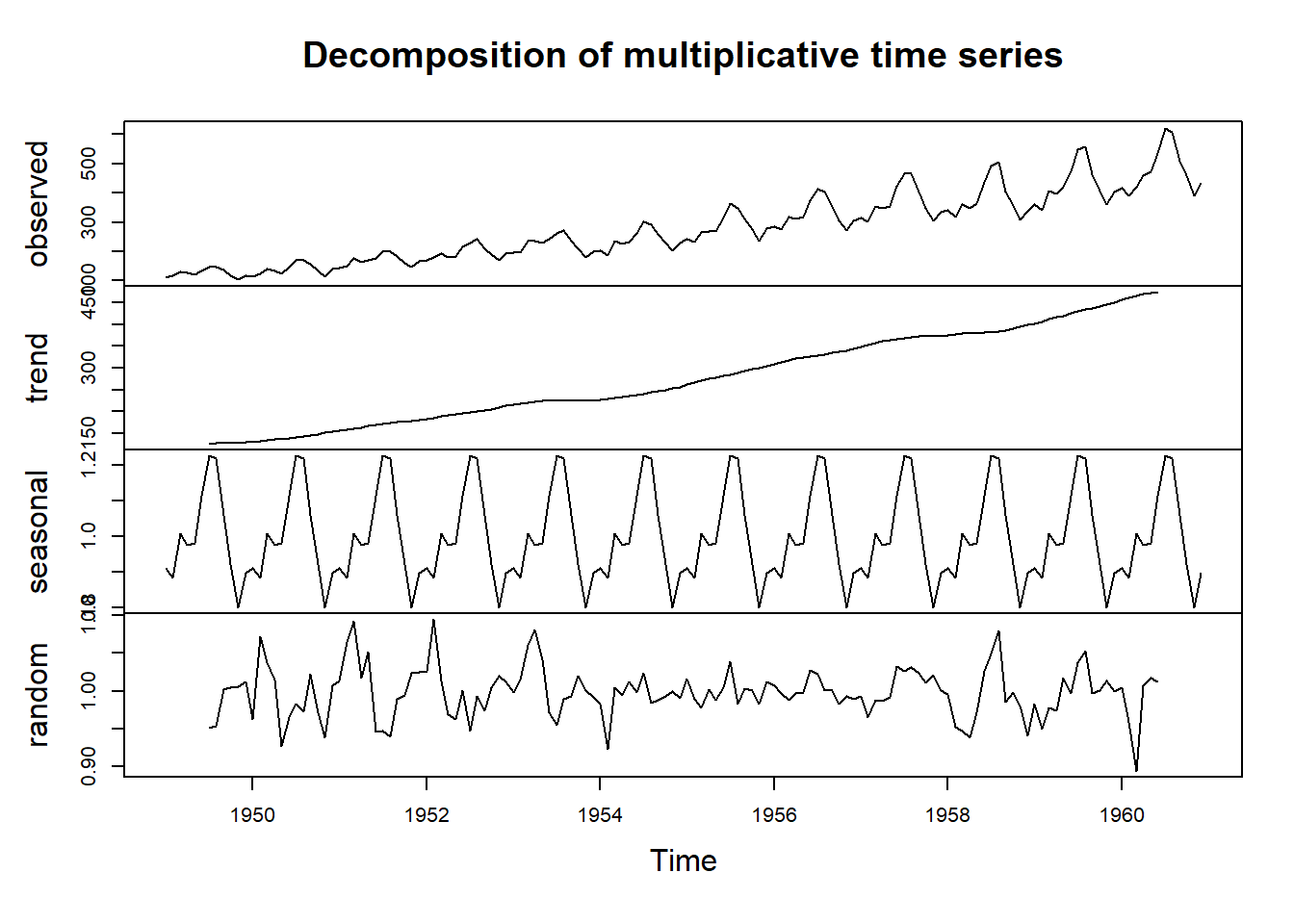ACF and PACF plots When choosing the order of p, d and q in the ARIMA models, ACF and PACF can help establish whether the series needs autoregressive terms (p), moving average terms (q). Also, ACF/PACF can also lend us a hand in deciding the order of integration (d) of the series. These plots usually give a good starting point in the application of ARIMA models. acf(tsdata)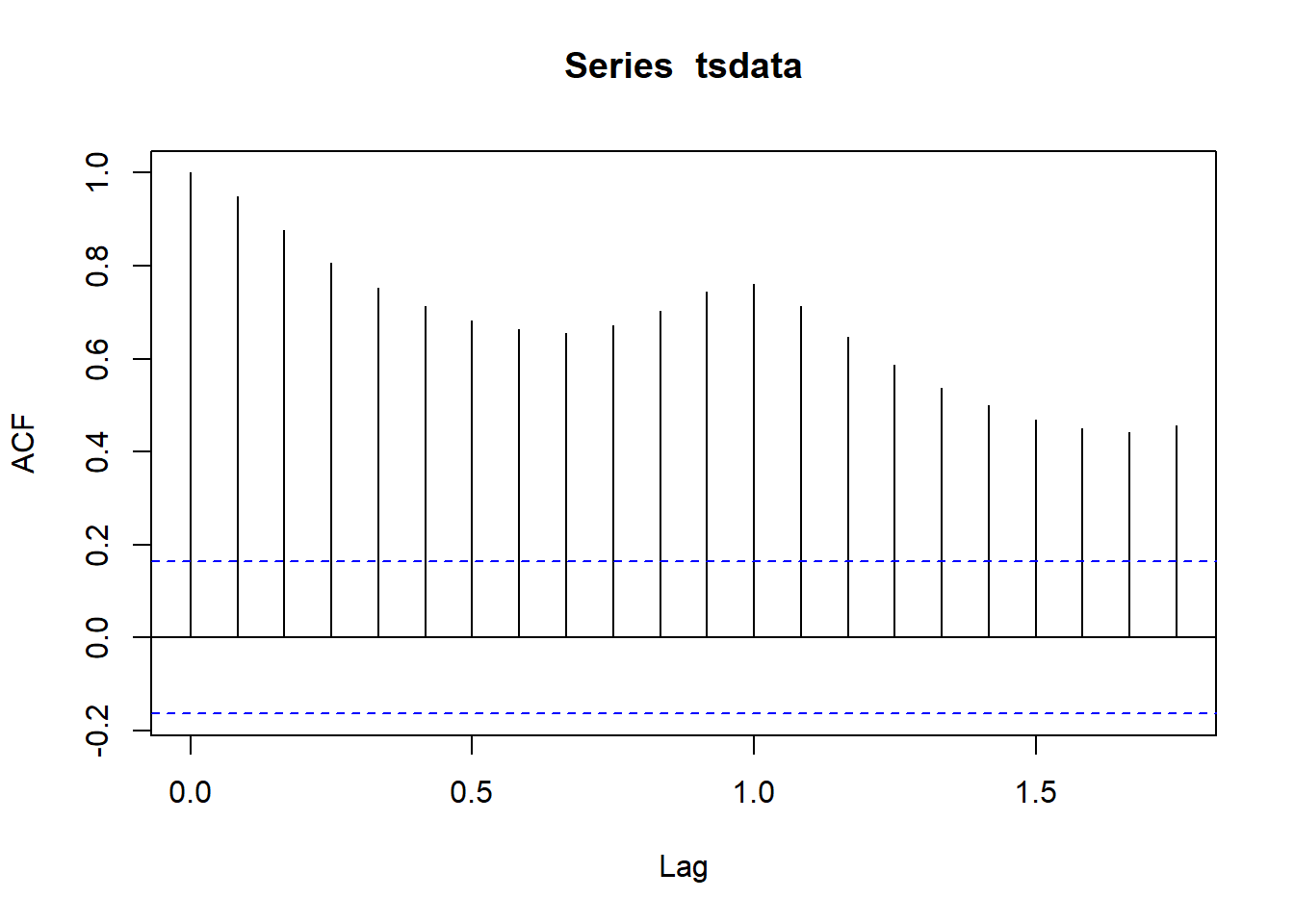pacf(tsdata)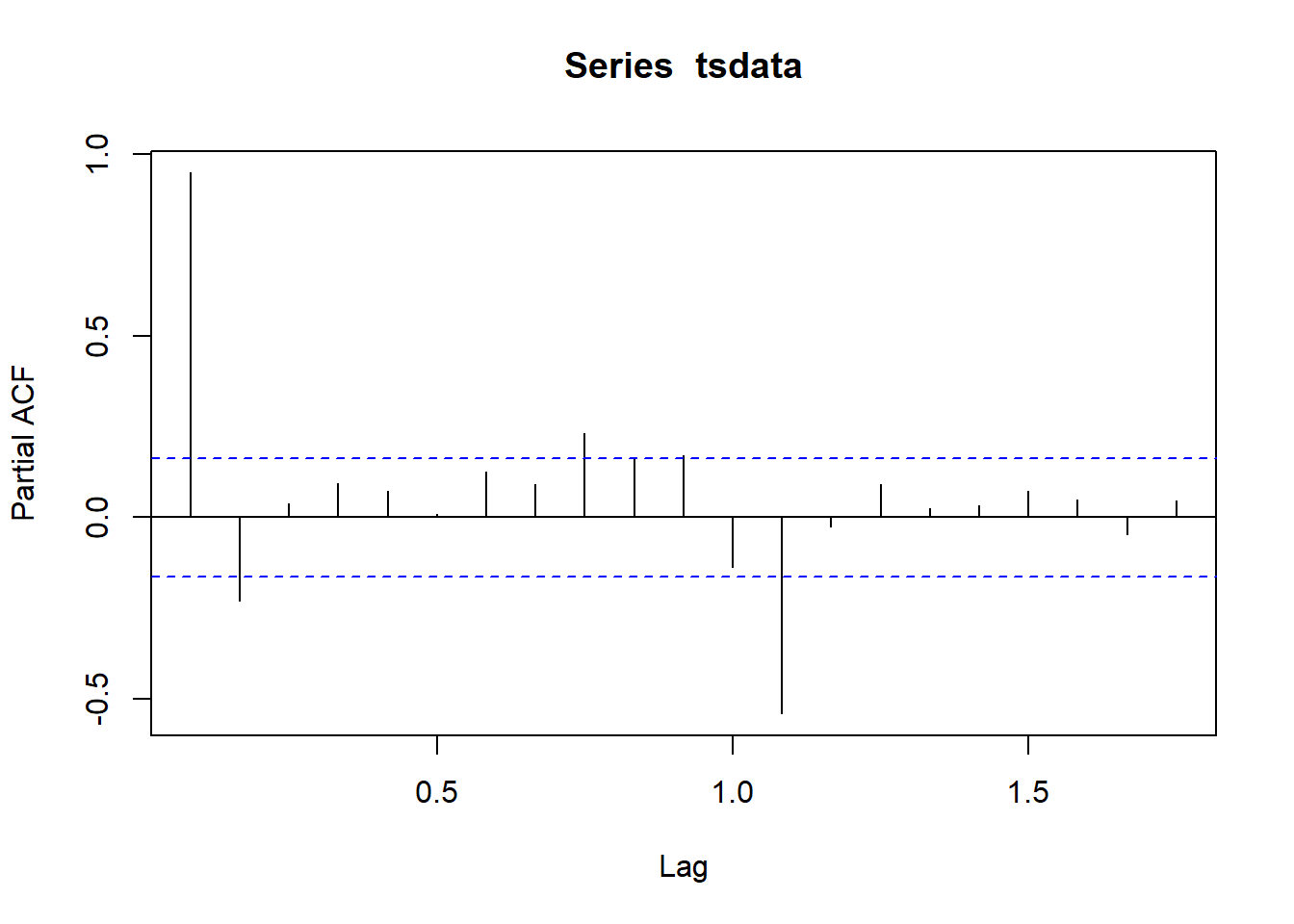acf(diff(tsdata))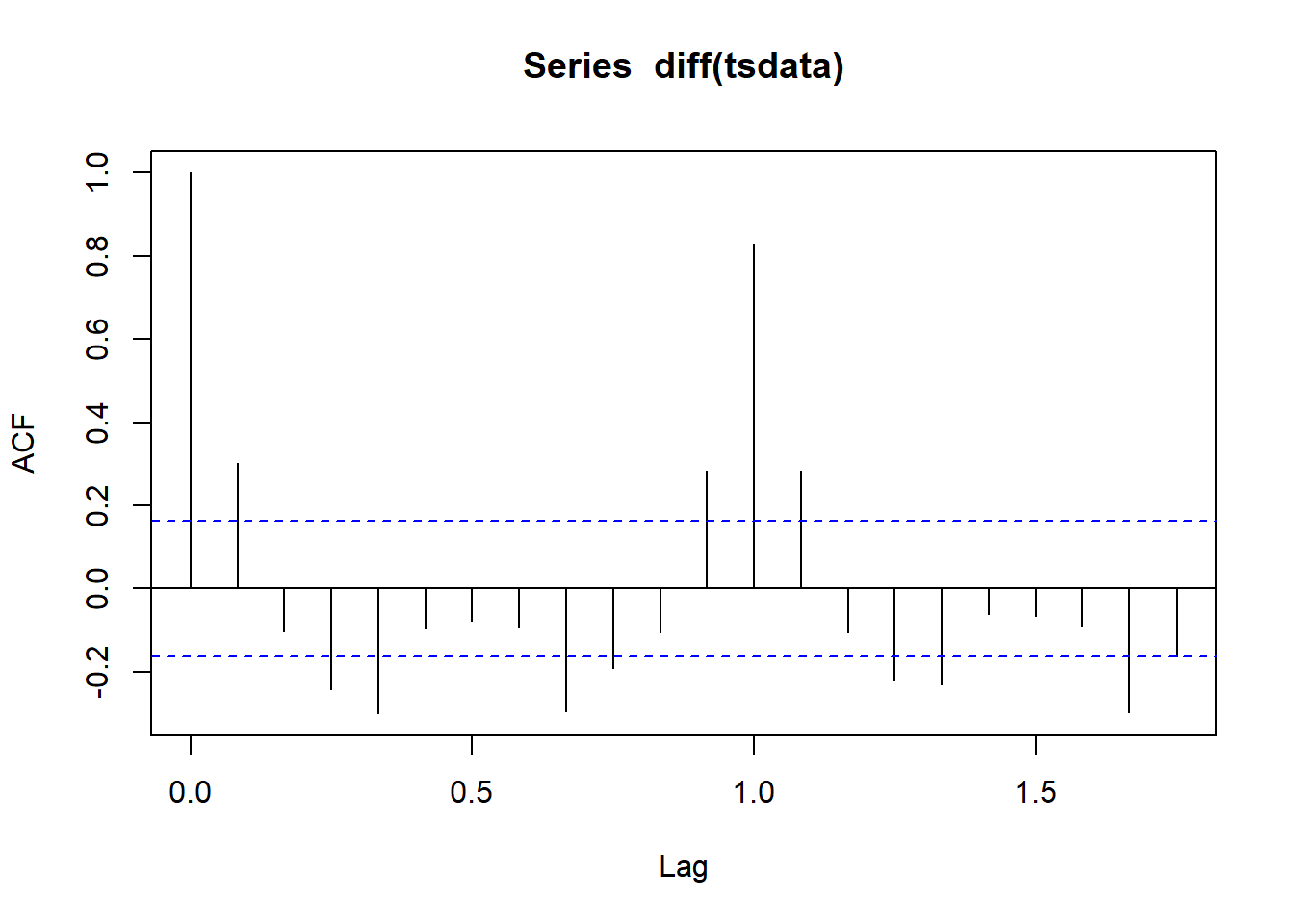pacf(diff(tsdata))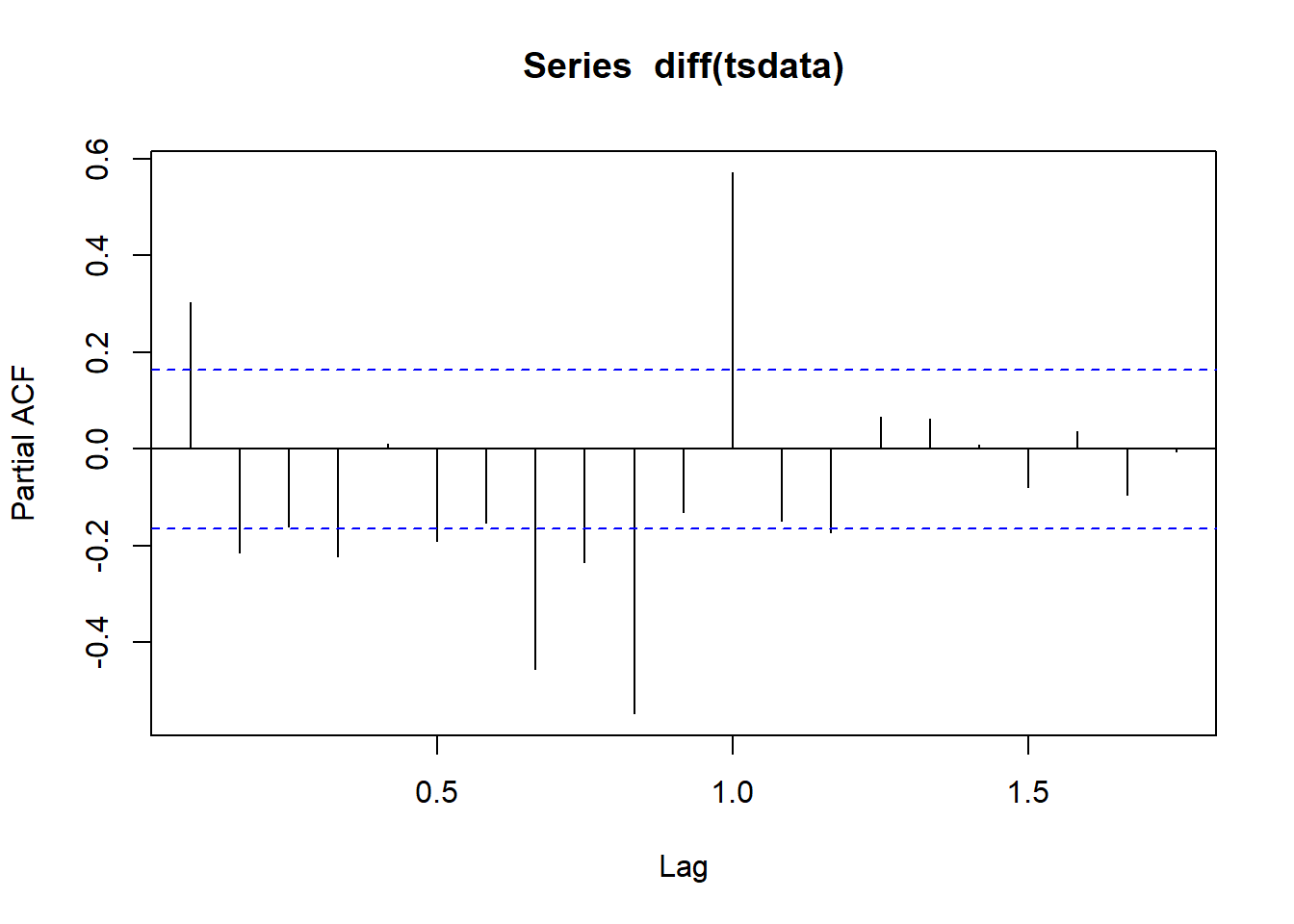Estimating ARIMA models There are several packages and functions that can estimate the ARIMA models. Here, we will use the “arima” function to estimate the model. This function allows us to specify a number of arguments for the model. Some of the most useful arguments are: 1. order = c(p,d,q): to specifiy the order of ARIMA(p,d,q) where ‘p’ is the number of autoregressive terms, ‘d’ is the order of differences and ‘q’ is the number of moving average terms. 2. xreg = data: to specify additional independent variables 3. seasonal = list(order = c(P,D,Q), period = frequency of season): this argument is used to specify the seasonal component when applying the SARIMA model. Here, ‘P’ is the number of seasonal autoregressive terms, ‘D’ is the order of seasonal differences and ‘Q’ is the number of seasonal moving average terms. 4. method = “CSS-ML”, “ML” or “CSS”: to choose the method used to estimate the ARIMA/SARIMA model where ‘ML’ refers to Maximum Likelihood estimation, ‘CSS’ refers to minimzing the Conditional Sum of Squares and ‘CSS-ML’ is a combination of both in which CSS is used to find starting values and switches to ML after that. arima110 <- arima(tsdata, order = c(1,1,0), xreg = NULL, include.mean = TRUE, init = NULL, method = "ML", optim.method = "BFGS") err <- resid(arima110) stargazer(arima110, type = "text", title = "ARIMA (1,1,0)", style = "all") ## ## ARIMA (1,1,0) ## ============================================= ## Dependent variable: ## --------------------------- ## tsdata ## --------------------------------------------- ## ar1 0.307*** ## (0.080) ## t = 3.848 ## p = 0.0002 ## --------------------------------------------- ## Observations 143 ## Log Likelihood -698.926 ## sigma2 1,029.292 ## Akaike Inf. Crit. 1,401.853 ## ============================================= ## Note: *p<0.1; **p<0.05; ***p<0.01 acf(err, lag.max = 36)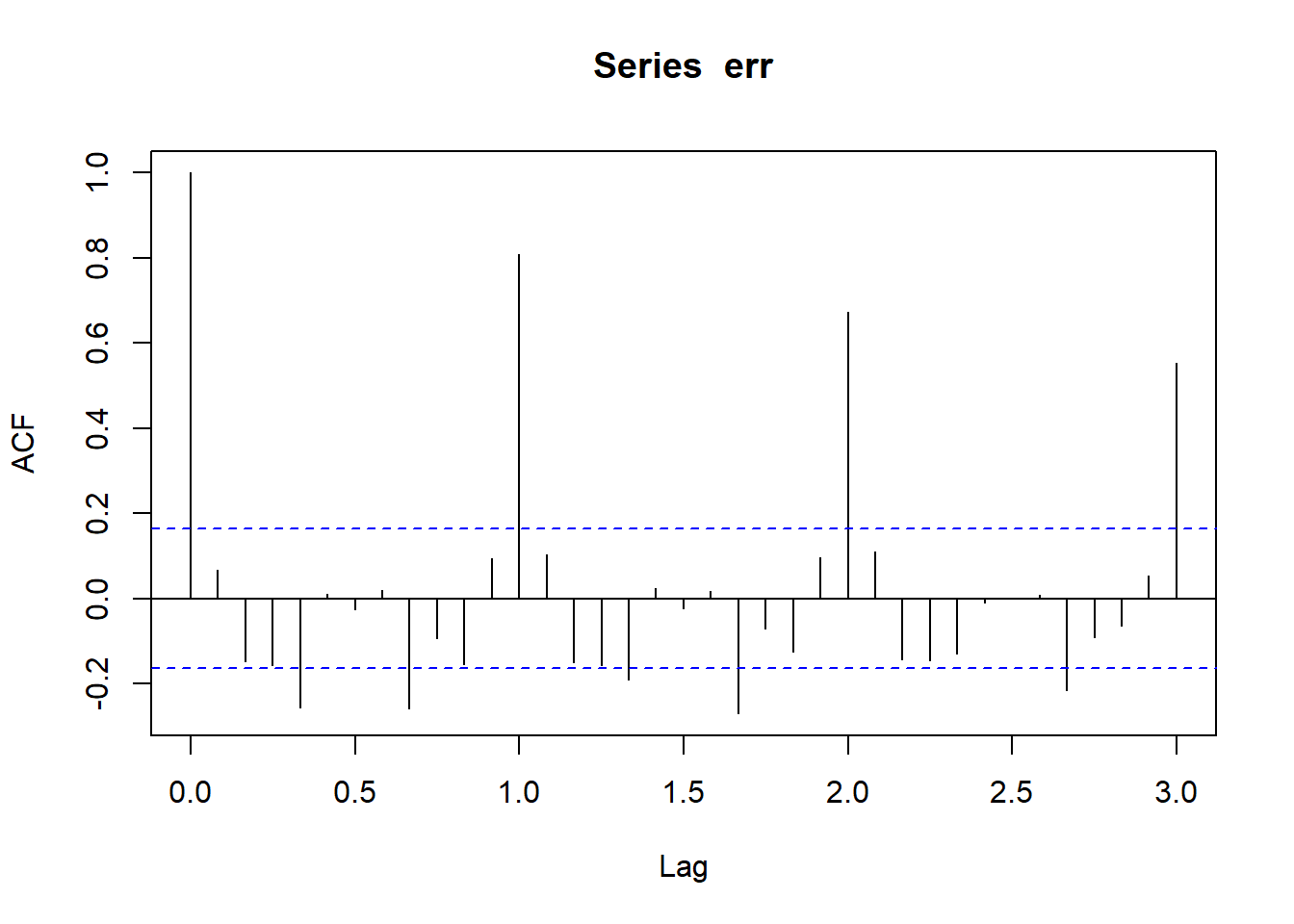pacf(err, lag.max = 36)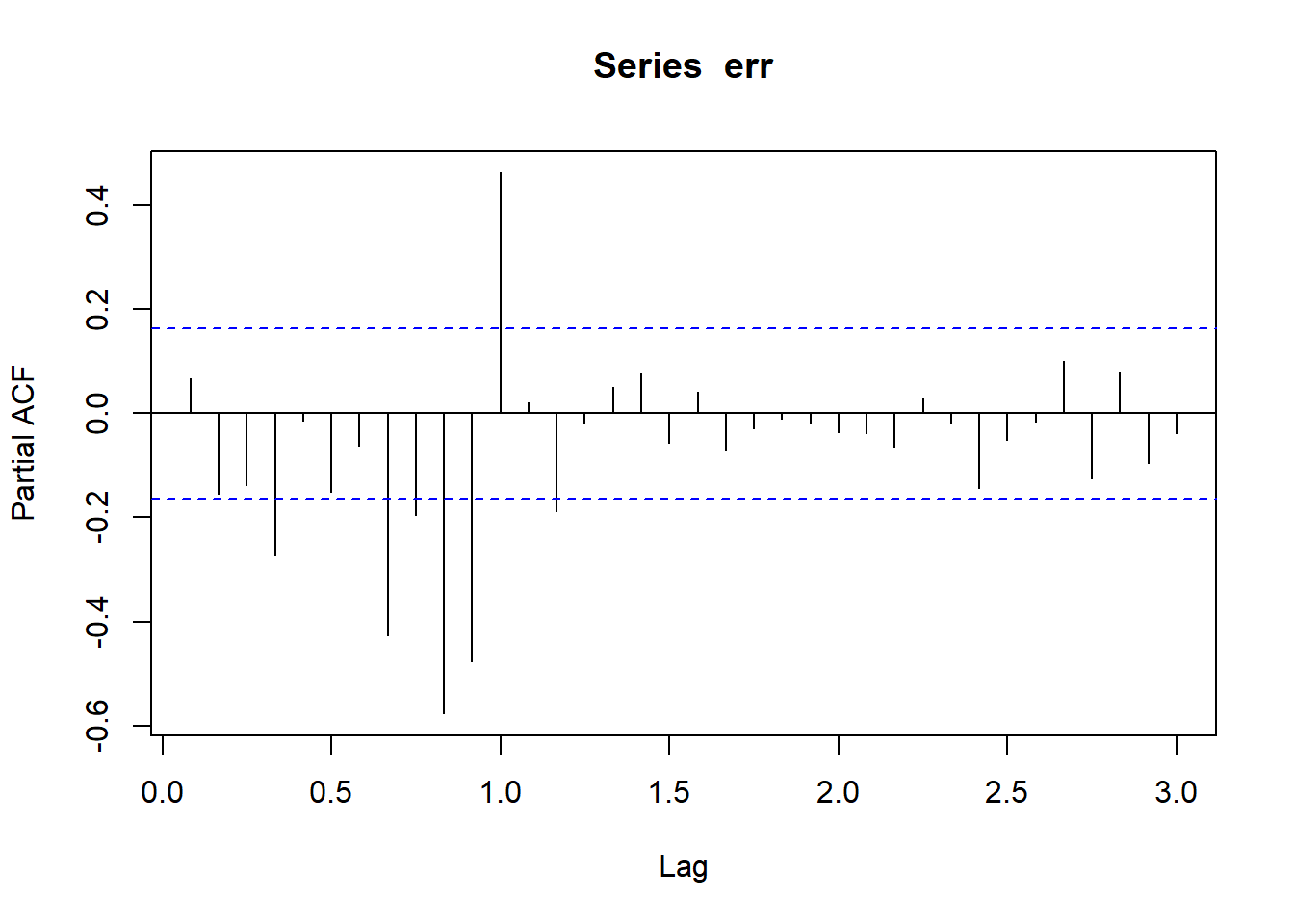Estimating SARIMA models sarima100 <- arima(tsdata, order = c(1,1,0), seasonal = list(order = c(1,0,0),period = 12), include.mean = FALSE, method = "ML") stargazer(sarima100, type = "text", title = "SARIMA (1,1,0)(1,0,0,12)", style = "all") ## ## SARIMA (1,1,0)(1,0,0,12) ## ============================================= ## Dependent variable: ## --------------------------- ## tsdata ## --------------------------------------------- ## ar1 -0.255*** ## (0.083) ## t = -3.060 ## p = 0.003 ## sar1 0.959*** ## (0.016) ## t = 61.816 ## p = 0.000 ## --------------------------------------------- ## Observations 143 ## Log Likelihood -568.941 ## sigma2 135.245 ## Akaike Inf. Crit. 1,143.883 ## ============================================= ## Note: *p<0.1; **p<0.05; ***p<0.01 sarima110 <- arima(tsdata, order = c(1,1,0), seasonal = list(order = c(1,1,0),period = 12), include.mean = FALSE, method = "ML") stargazer(sarima110, type = "text", title = "SARIMA (1,1,0)(1,1,0,12)", style = "all") ## ## SARIMA (1,1,0)(1,1,0,12) ## ============================================= ## Dependent variable: ## --------------------------- ## tsdata ## --------------------------------------------- ## ar1 -0.297*** ## (0.083) ## t = -3.551 ## p = 0.0004 ## sar1 -0.140 ## (0.098) ## t = -1.422 ## p = 0.155 ## --------------------------------------------- ## Observations 131 ## Log Likelihood -507.197 ## sigma2 134.703 ## Akaike Inf. Crit. 1,020.393 ## ============================================= ## Note: *p<0.1; **p<0.05; ***p<0.01 err_sarima110 <- resid(sarima110) acf(err_sarima110, lag.max = 36)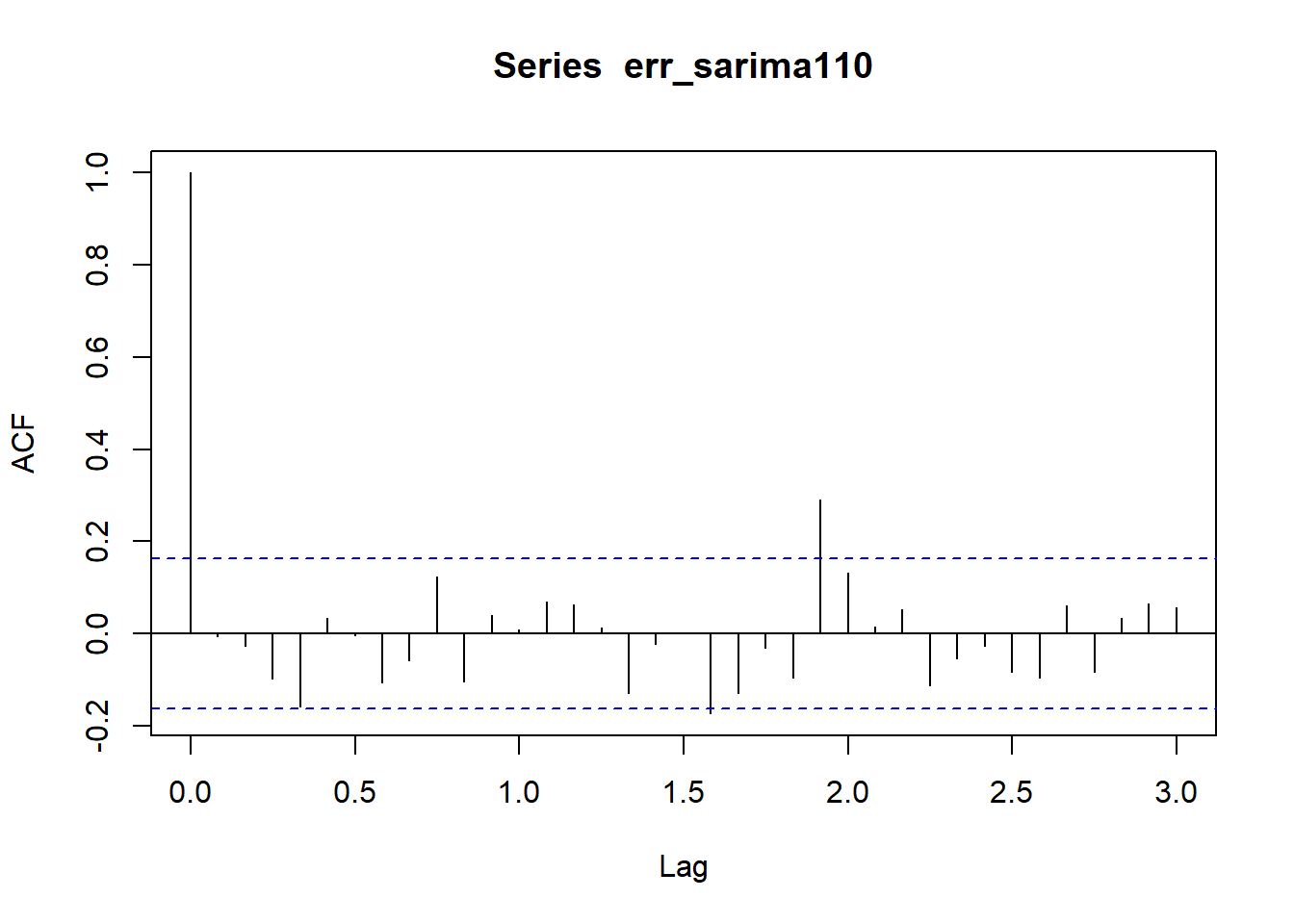pacf(err_sarima110, lag.max = 36)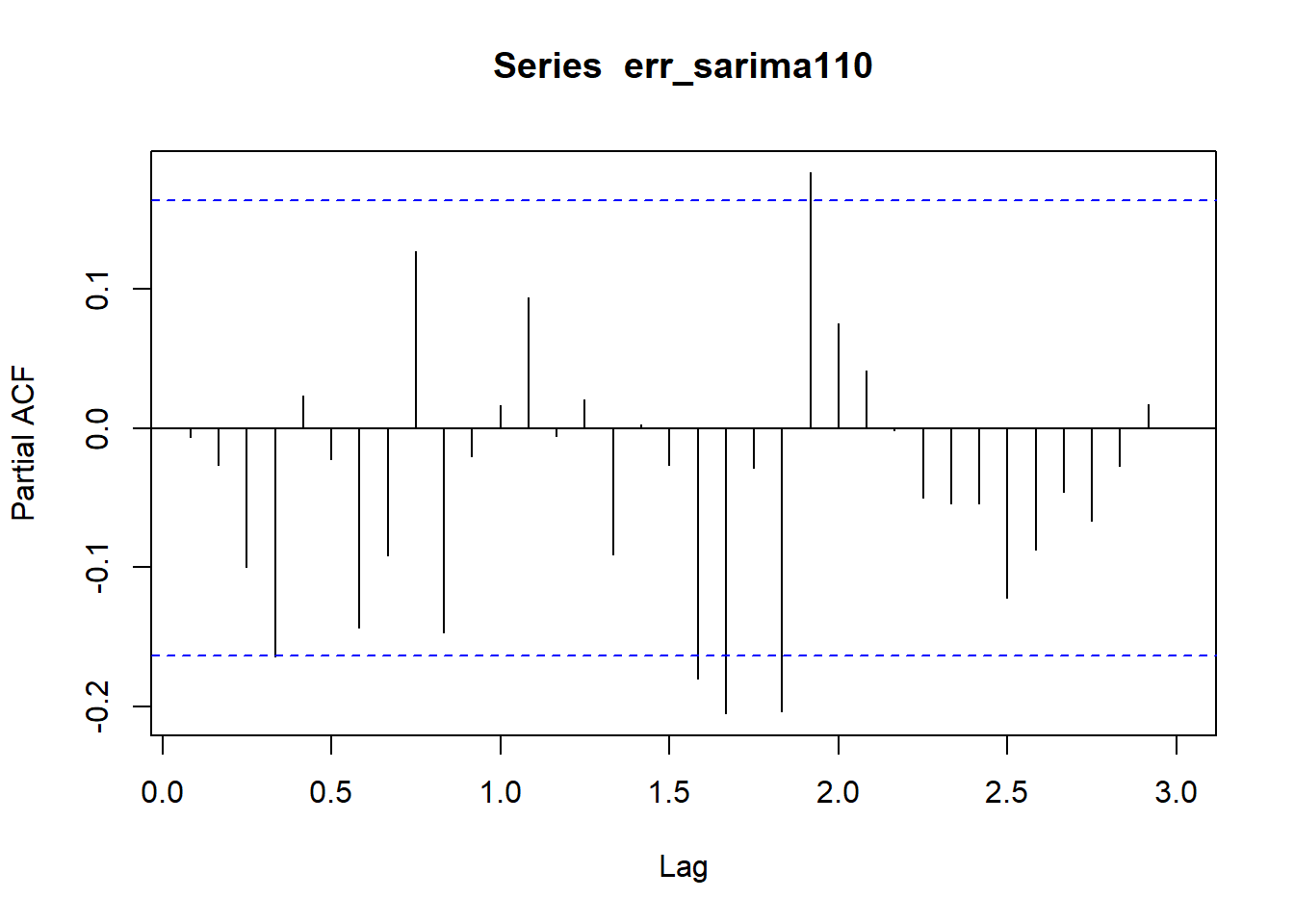Another example: SARIMA (1,1,0)(1,1,1,12) sarima111 <- arima(tsdata, order = c(1,1,0), seasonal = list(order = c(1,1,1),period = 12), include.mean = FALSE, method = "ML") stargazer(sarima111, type = "text", title = "SARIMA (1,1,0)(1,1,1,12)", style = "all") ## ## SARIMA (1,1,0)(1,1,1,12) ## ============================================= ## Dependent variable: ## --------------------------- ## tsdata ## --------------------------------------------- ## ar1 -0.323*** ## (0.083) ## t = -3.907 ## p = 0.0001 ## sar1 -0.893*** ## (0.276) ## t = -3.234 ## p = 0.002 ## sma1 0.794** ## (0.382) ## t = 2.076 ## p = 0.038 ## --------------------------------------------- ## Observations 131 ## Log Likelihood -506.247 ## sigma2 131.506 ## Akaike Inf. Crit. 1,020.493 ## ============================================= ## Note: *p<0.1; **p<0.05; ***p<0.01 err_sarima111 <- resid(sarima111) acf(err_sarima111, lag.max = 36)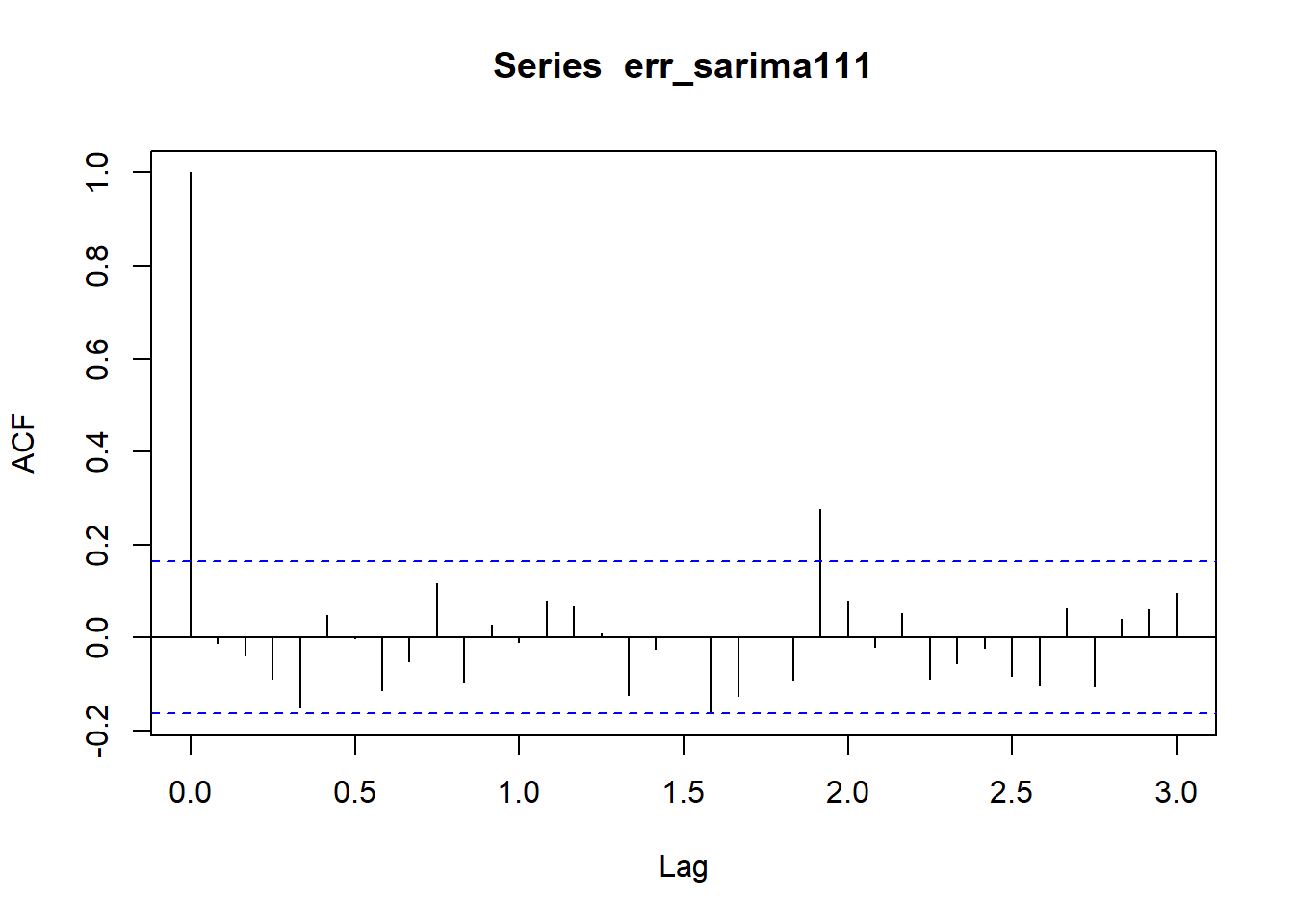pacf(err_sarima111, lag.max = 36)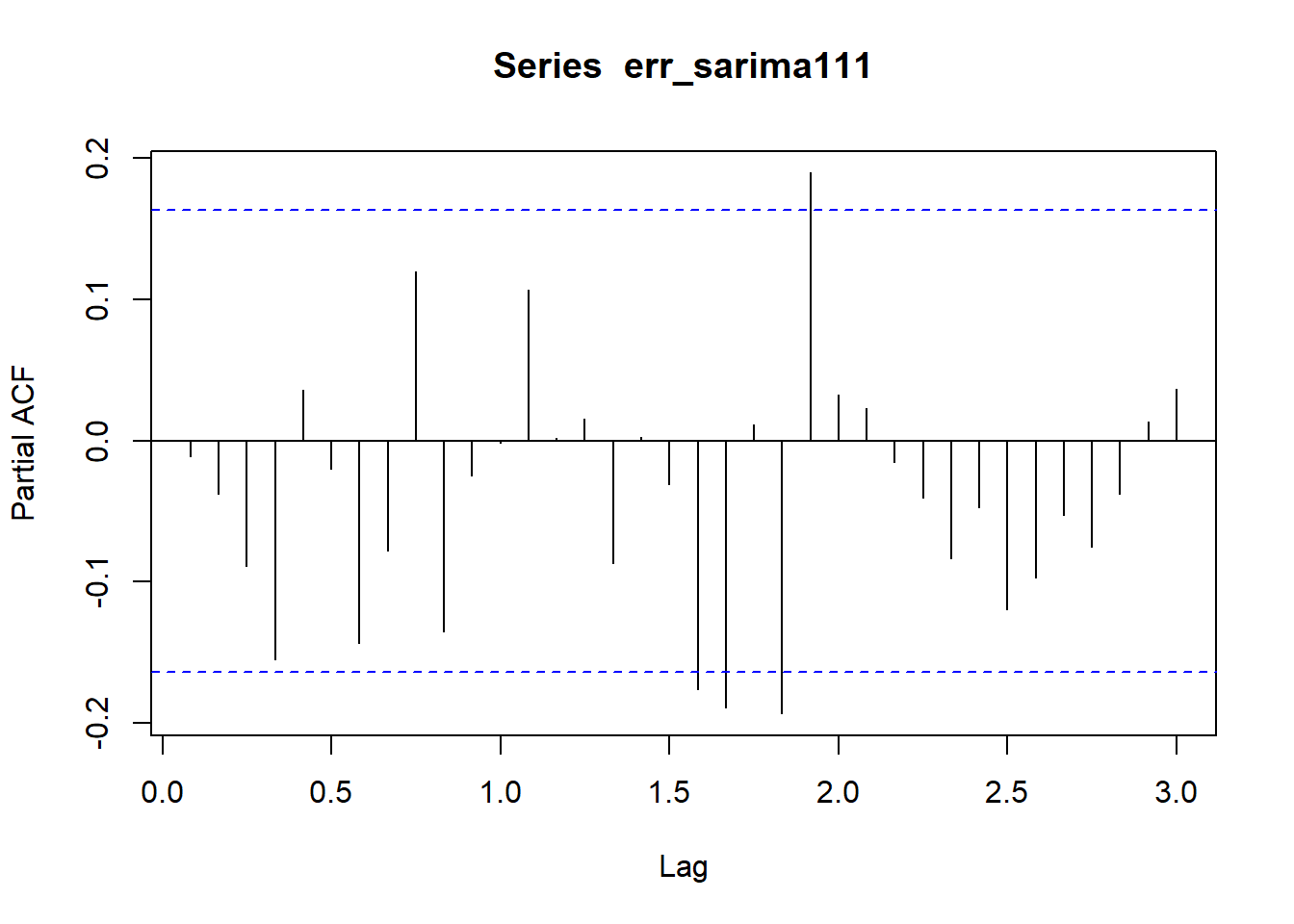Forecasting Usually, the ARIMA and SARIMA models are used for forecasting purposes and they have been observed to perform well. One of the best uses of these models is to predict or forecast into the future. forecast_sarima111 <- predict(sarima111, n.ahead = 36, newxreg = NULL) forecast_s111 <- forecast(sarima111, h = 36) ts.plot(tsdata, forecast_sarima111$pred)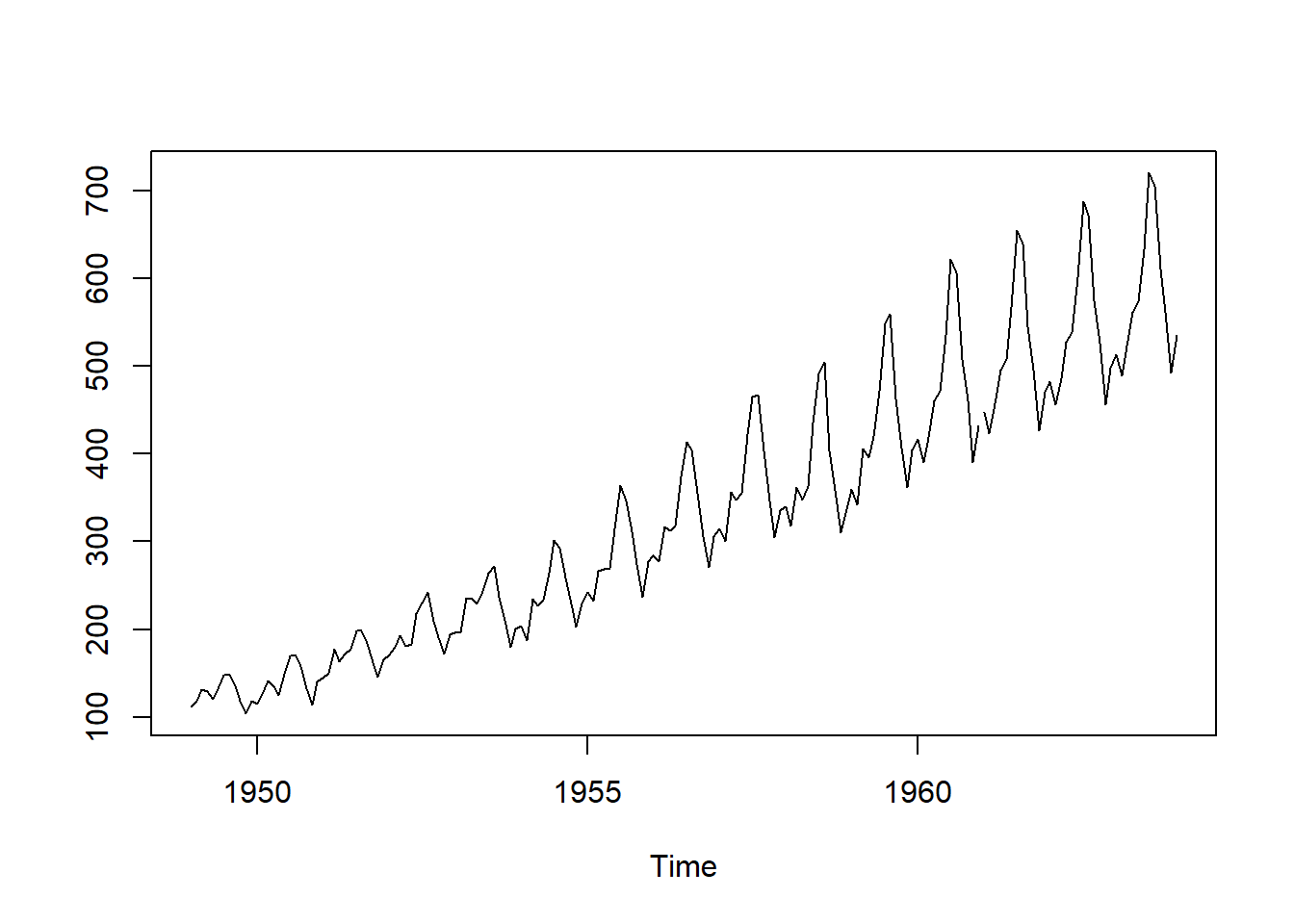plot(forecast_s111)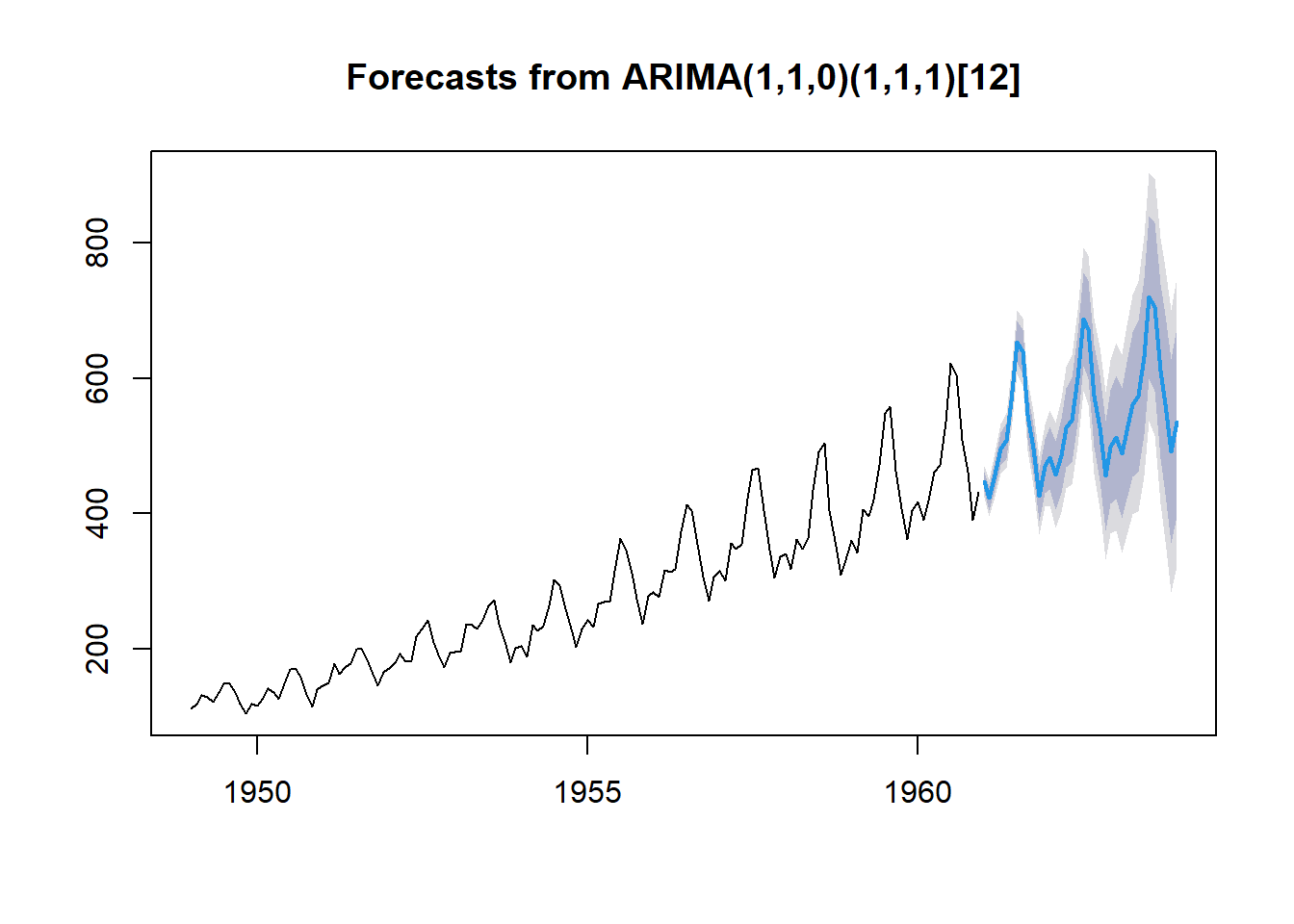Automatic estimation of ARIMA and SARIMA: auto.arima

The “auto.arima” function can be used to estimate ARIMA and SARIMA models. This function automatically chooses the order of the model, performs stationarity tests and chooses the best model with the help of Information Criteria (AIC, BIC or AICC).

This function performs an iterative procedure to estimate several ARIMA or SARIMA models with different orders of ARIMA(p,d,q) and SARIMA(P,D,Q). Then, it chooses the model With help of AIC, BIC or AICC. The model that minimzes the information criteria is chosen and its results are reported.

autoarima <- auto.arima(tsdata, trace = TRUE)
##
##  ARIMA(2,1,2)(1,1,1)                    : Inf
##  ARIMA(0,1,0)(0,1,0)                    : 1031.539
##  ARIMA(1,1,0)(1,1,0)                    : 1020.582
##  ARIMA(0,1,1)(0,1,1)                    : 1021.192
##  ARIMA(1,1,0)(0,1,0)                    : 1020.488
##  ARIMA(1,1,0)(0,1,1)                    : 1021.103
##  ARIMA(1,1,0)(1,1,1)                    : Inf
##  ARIMA(2,1,0)(0,1,0)                    : 1022.583
##  ARIMA(1,1,1)(0,1,0)                    : 1022.583
##  ARIMA(0,1,1)(0,1,0)                    : 1020.733
##  ARIMA(2,1,1)(0,1,0)                    : 1018.165
##  ARIMA(2,1,1)(1,1,0)                    : 1018.395
##  ARIMA(2,1,1)(0,1,1)                    : 1018.84
##  ARIMA(2,1,1)(1,1,1)                    : Inf
##  ARIMA(3,1,1)(0,1,0)                    : 1019.565
##  ARIMA(2,1,2)(0,1,0)                    : 1019.771
##  ARIMA(1,1,2)(0,1,0)                    : 1024.478
##  ARIMA(3,1,0)(0,1,0)                    : 1023.984
##  ARIMA(3,1,2)(0,1,0)                    : Inf
##
##  Best model: ARIMA(2,1,1)(0,1,0)
autoarima
## Series: tsdata
## ARIMA(2,1,1)(0,1,0)
##
## Coefficients:
##          ar1     ar2      ma1
##       0.5960  0.2143  -0.9819
## s.e.  0.0888  0.0880   0.0292
##
## sigma^2 = 132.3:  log likelihood = -504.92
## AIC=1017.85   AICc=1018.17   BIC=1029.35
plot(forecast(autoarima, h = 36))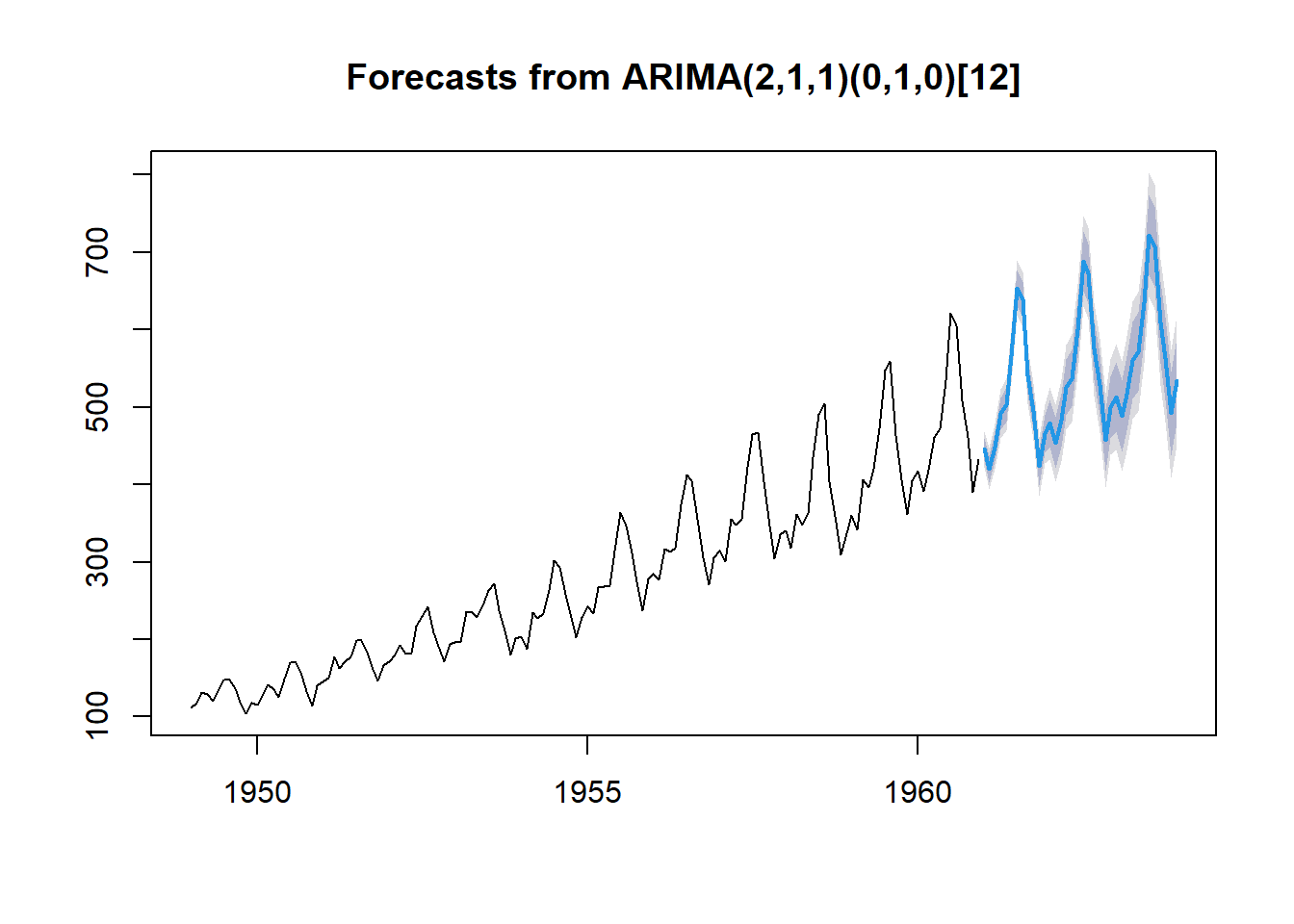Further commands in auto.arima

The “auto.arima” function provides a larger number of arguments. Some of the important ones are:

1. stationary = TRUE: only stationary models are considered

2. seasonal = FALSE: only non-seasonal models are considered

3. ic = “aic”, “bic” or “aicc”: information criteria to minimize

4. trace = TRUE: report all the models that were considered (only order and information criteria are reported)

5. allowdrift = TRUE: models with drift are included

6. allowmean = TRUE: models with non-zero mean are included

7. xreg and method: similar to the previous “arima” command

autoarima1 <- auto.arima(tsdata,
trace = TRUE,
ic = "aic",
method = "ML",
test = "kpss",
allowdrift = TRUE)
##
##  ARIMA(2,1,2)(1,1,1)                    : 1020.049
##  ARIMA(0,1,0)(0,1,0)                    : 1031.508
##  ARIMA(1,1,0)(1,1,0)                    : 1020.393
##  ARIMA(0,1,1)(0,1,1)                    : 1021.003
##  ARIMA(2,1,2)(0,1,1)                    : 1019.936
##  ARIMA(2,1,2)(0,1,0)                    : 1019.291
##  ARIMA(2,1,2)(1,1,0)                    : 1019.547
##  ARIMA(1,1,2)(0,1,0)                    : 1024.161
##  ARIMA(2,1,1)(0,1,0)                    : 1017.848
##  ARIMA(2,1,1)(1,1,0)                    : 1017.915
##  ARIMA(2,1,1)(0,1,1)                    : 1018.36
##  ARIMA(2,1,1)(1,1,1)                    : Inf
##  ARIMA(1,1,1)(0,1,0)                    : 1022.394
##  ARIMA(2,1,0)(0,1,0)                    : 1022.394
##  ARIMA(3,1,1)(0,1,0)                    : 1019.085
##  ARIMA(1,1,0)(0,1,0)                    : 1020.394
##  ARIMA(3,1,0)(0,1,0)                    : 1023.666
##  ARIMA(3,1,2)(0,1,0)                    : 1021.084
##
##  Best model: ARIMA(2,1,1)(0,1,0)
autoarima1
## Series: tsdata
## ARIMA(2,1,1)(0,1,0)
##
## Coefficients:
##          ar1     ar2      ma1
##       0.5960  0.2143  -0.9819
## s.e.  0.0888  0.0880   0.0292
##
## sigma^2 = 132.3:  log likelihood = -504.92
## AIC=1017.85   AICc=1018.17   BIC=1029.35
plot(forecast(autoarima1, h = 36))The “auto.arima” command seems like a great option in every situation. However, it may not always choose the best model. For example, it uses only Information Criteria to choose the model and we might end up choosing a model with autocorrelated residuals. If the purpose is forecasting, it might be better to choose the model with help of forecasting performance instead. Still, it can be an extremely useful function.